Prime and Composite Numbers

Chapter 3 Class 6 Playing with Numbers
Concept wise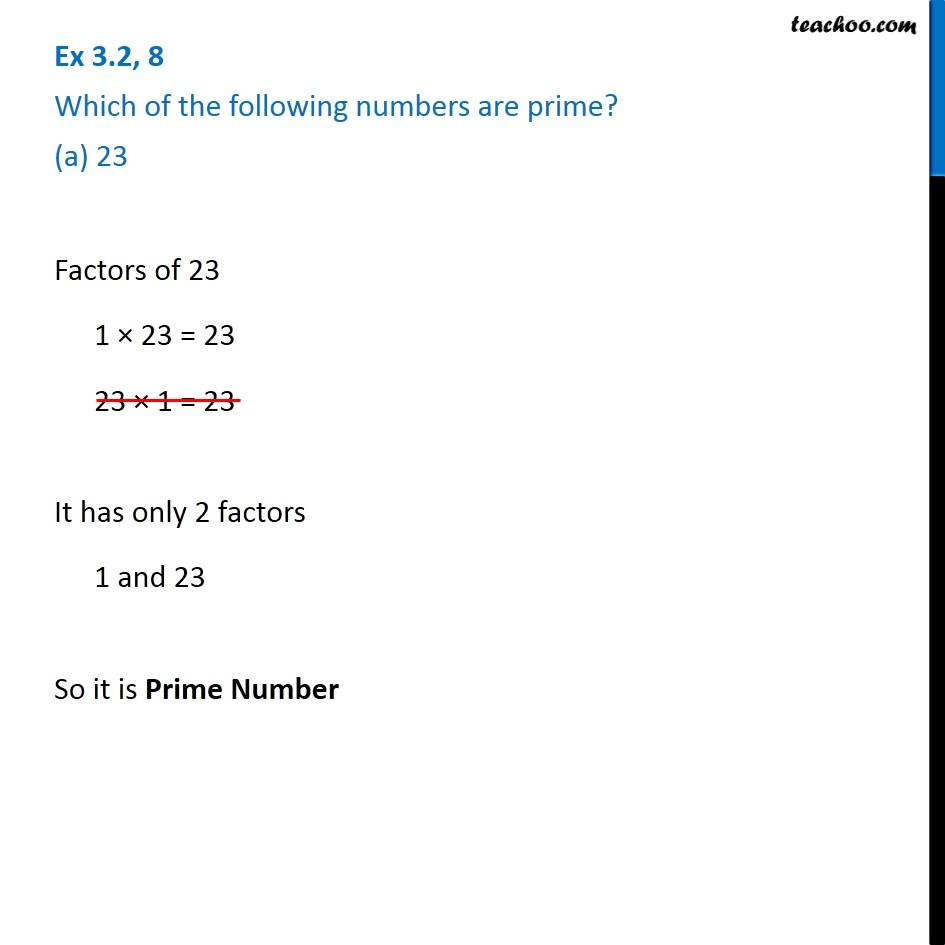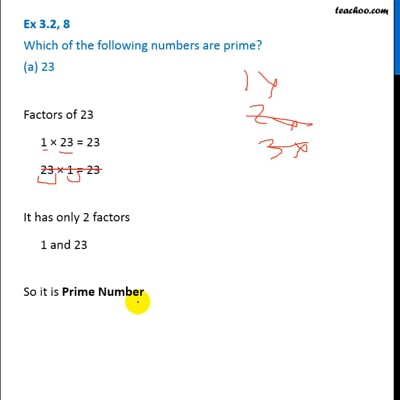This video is only available for Teachoo black users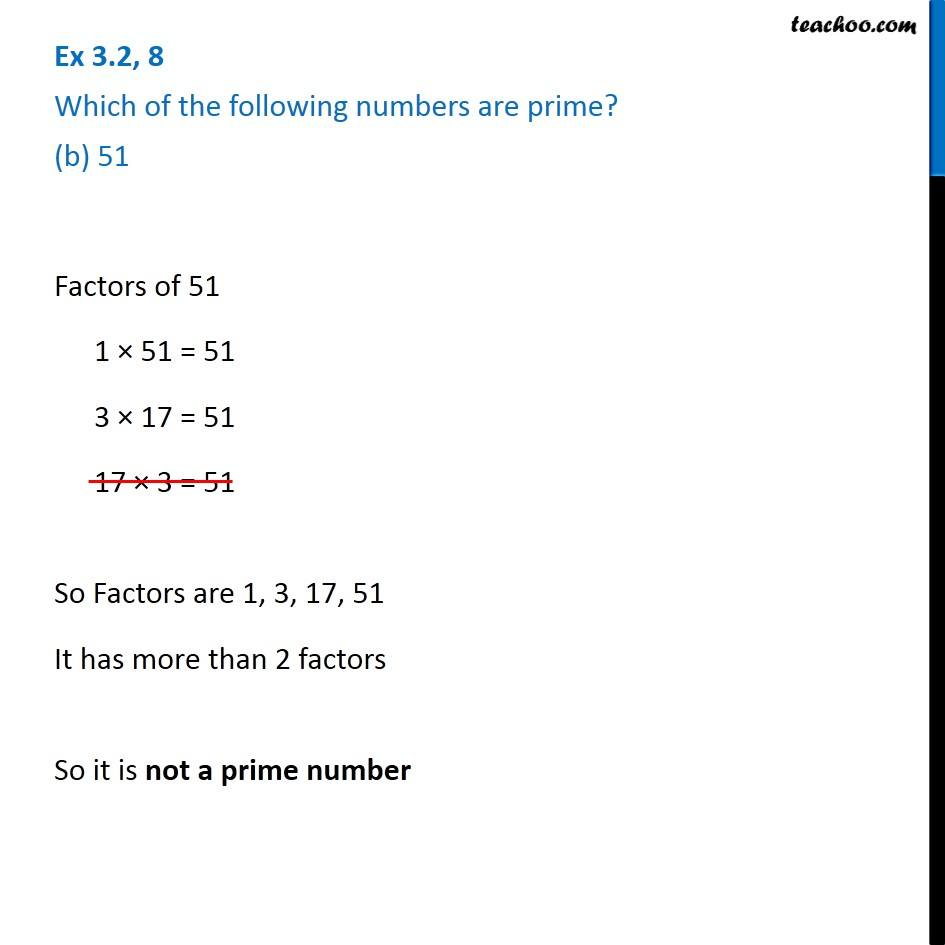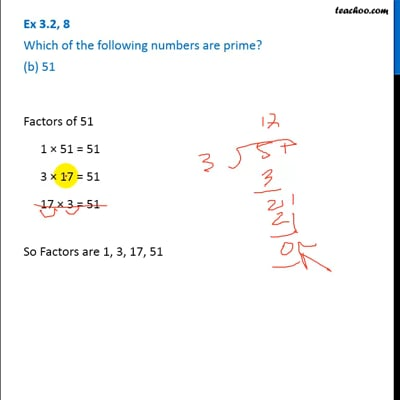This video is only available for Teachoo black users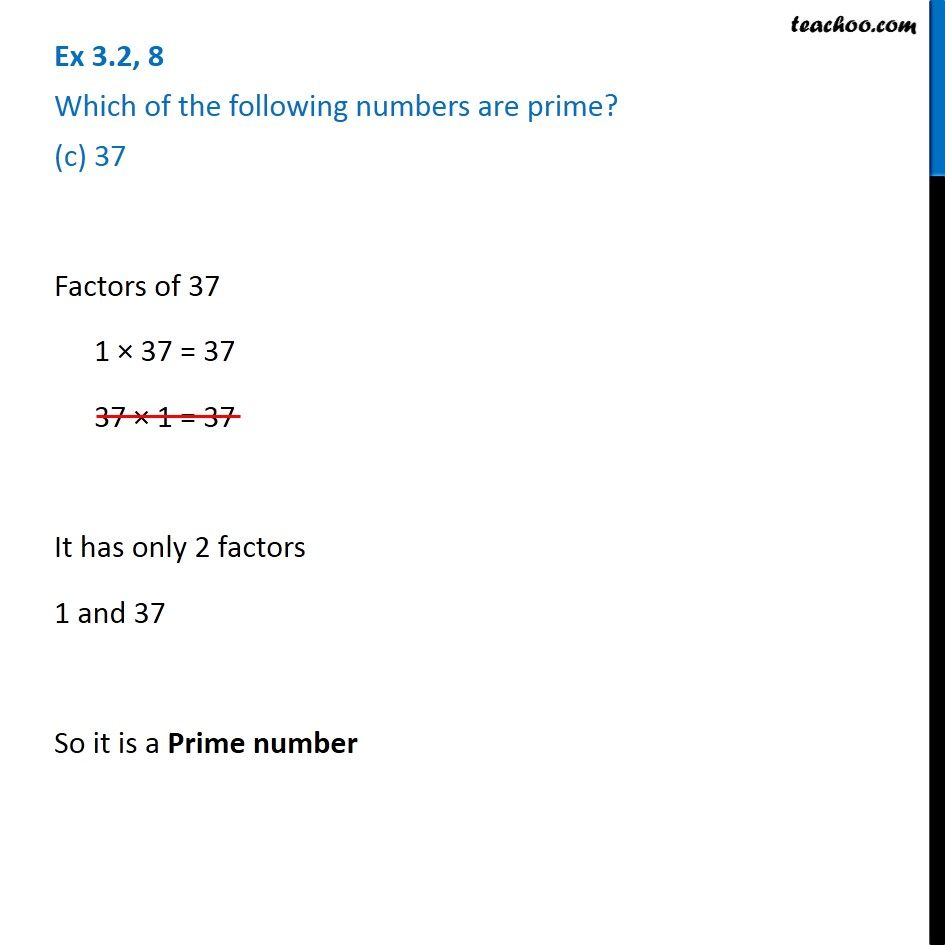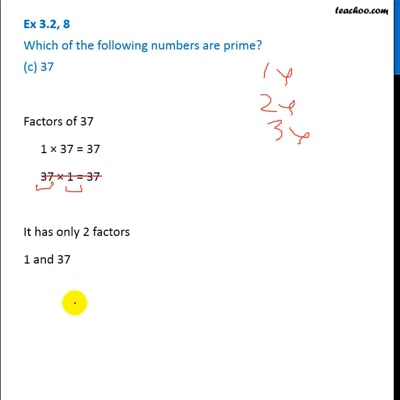This video is only available for Teachoo black users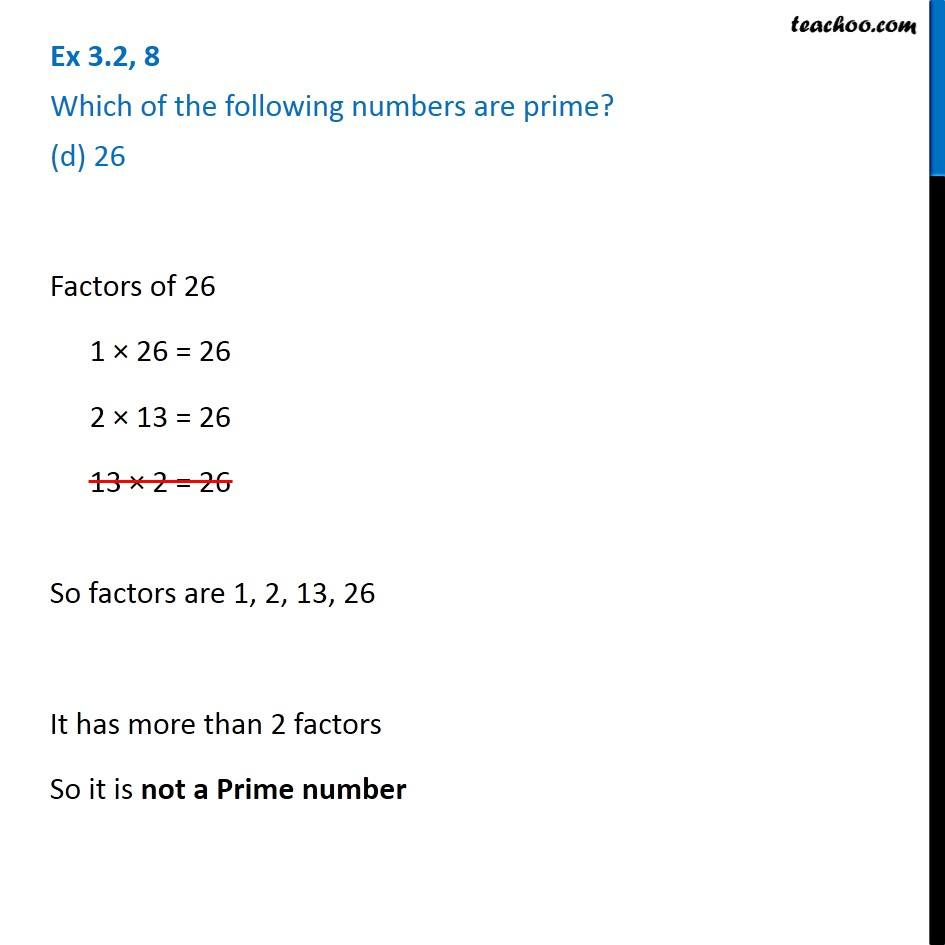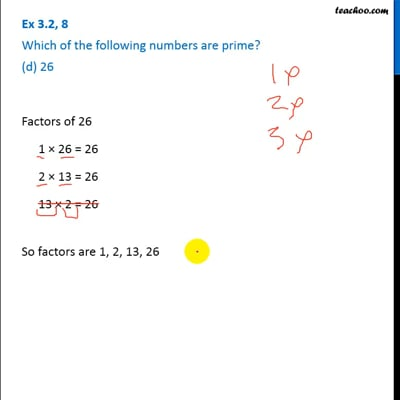This video is only available for Teachoo black users

Introducing your new favourite teacher - Teachoo Black, at only ₹83 per month

### Transcript

Ex 3.2, 8 Which of the following numbers are prime? (a) 23Factors of 23 1 × 23 = 23 23 × 1 = 23 It has only 2 factors 1 and 23 So it is Prime Number Ex 3.2, 8 Which of the following numbers are prime? (b) 51Factors of 51 1 × 51 = 51 3 × 17 = 51 17 × 3 = 51 So Factors are 1, 3, 17, 51 It has more than 2 factors So it is not a prime number Ex 3.2, 8 Which of the following numbers are prime? (c) 37Factors of 37 1 × 37 = 37 37 × 1 = 37 It has only 2 factors 1 and 37 So it is a Prime number Ex 3.2, 8 Which of the following numbers are prime? (d) 26Factors of 26 1 × 26 = 26 2 × 13 = 26 13 × 2 = 26 So factors are 1, 2, 13, 26 It has more than 2 factors So it is not a Prime number